# MCQ Relations and Functions

The domain of definition of the functionis
(A) [1,4]
(B) [1,0]
(C) [0,5]
(D) [5,0]

Solution: (A)
Given thatFor domain of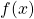,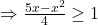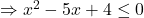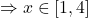Letbe a relation on the set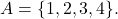The relation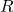is
(A) a function
(B) transitive
(C) not symmetric
(D) reflexive

Solution: (C)
Since,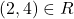and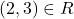So, R is not a function.
Since,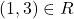and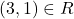but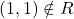So, R is not transitive
Since,but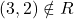so, R is not symmetric
Since,so, R is not reflexive

The domain of the function(A)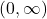(B)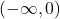(B)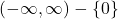(B)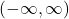Explanation:
We must have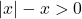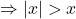This is only possible when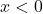Let R be a relation defined as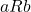if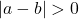. Then, the relationis
(
A) Reflexive
(B) Symmetric
(C) Transitive
(D) None of theseis not reflexive since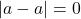is symmetric since if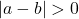, then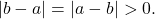Thus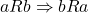since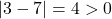and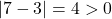, so we have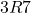and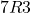But 3 is not related to 3 as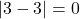.